# Cost Management

## Quiz 6 : Process CostingStudy FlashcardsLooking for Management Homework Help?

## Quiz 6 :Process Costing

Question TypeHow is the equivalent unit calculation affected when direct materials are added at the beginning of the process rather than uniformly throughout the process
Free
Essay

Equivalent units:
In a manufacturing concern production is a continuous process. But at the end of the accounting period every organization is required to calculate the cost incurred in production but it may cause problem due to the work in progress in the inventory.
Wok-in-progress inventory means that raw material on which process has started but has not been completed yet. Hence, for the sake of calculating cost companies convert the partially completed units into completed units which are known as the equivalent units.
Direct Material:
Direct materials are those raw materials which are used directly in the manufacturing process. These are the key raw material for the final output. Manufacturing process which requires more than one process use calculation of equivalent units.
Direct materials can be added at the beginning of production process or throughout the production process as per the requirement.
Equivalent units when direct materials are added at the beginning of the period:
When equivalent units are added at the beginning of the period, than it is assumed that it will be completed 100% by the end of the period hence, equivalent units will be equal to the units added at the beginning of the period.
When direct material is added throughout the production process than equivalent units will be equal to the completion of conversion cost percentage.

Tags
Choose question tag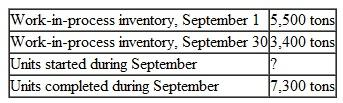Free
Essay

Work in progress:
Work in progress in a manufacturing concern refers to the partially finished goods on which work will be completed in the next production period.
Production process is a continuous process and organization require to calculation cost at the end of period to estimate the cost of the product. Because of the continuous production it is necessary that few units are partially completed and will be completed in the next year. These units are known as work in progress.
Units started during the period:
Units started during the period means those units which are started afresh in the current period. i.e. on this units no work is done in the prior period and these are not a part of Work in progress in the beginning of a period.
Units started in a year are calculated as follows: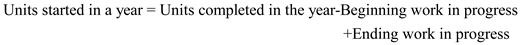Putting the values in the formula:Tags
Choose question tag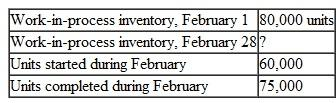Free
Essay

Work in progress:
Work in progress in a manufacturing concern refers to the partially finished goods on which work will be completed in the next production period.
Production process is a continuous process and organization require to calculation cost at the end of period to estimate the cost of the product. Because of the continuous production it is necessary that few units are partially completed and will be completed in the next year. These units are known as Work in progress.
Beginning Work in Progress:
Beginning work in progress are units which are incomplete at the beginning of the production period and will be completed in the current period.
Ending Work in Progress:
Ending work in progress are units which are incomplete at the end of the production period and will be completed in the next period.
It is calculated as follows: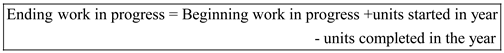Putting the values in the formulaTags
Choose question tag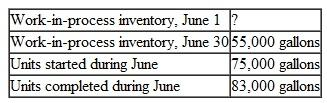Essay
Tags
Choose question tagWhat is the main difference between journal entries in process costing and in job costing
Essay
Tags
Choose question tagIdentify the conditions under which the weighted-average method of process costing is inappropriate.
Essay
Tags
Choose question tagSuppose that manufacturing is performed in sequential production departments. Prepare a journal entry to show a transfer of partially completed units from the first department to the second department. Assume the amount of cost transferred is \$50,000.
Essay
Tags
Choose question tagSpecify the advantage of the weighted-average method of process costing in contrast to the FIFO method.
Essay
Tags
Choose question tagWhat are transferred-in costs
Essay
Tags
Choose question tagWhat does the term equivalent units mean
Essay
Tags
Choose question tagFrom the standpoint of cost control, why is the FIFO method superior to the weighted-average method Is it possible to monitor cost trends using the weighted-average method
Essay
Tags
Choose question tagWhat is the difference between process costing and backflush costing
Essay
Tags
Choose question tagWhat is a production cost report What are the five key steps in preparing a production cost report
Essay
Tags
Choose question tagExplain the primary differences between job costing and process costing.
Essay
Tags
Choose question tagUnder the weighted-average method, all units transferred out are treated the same way. How does this differ from the FIFO method of handling units transferred out
Essay
Tags
Choose question tagUnder the FIFO method, only current period costs and work are included in unit costs and equivalent units computation. Under the weighted-average method, what assumptions are made when unit costs and equivalent units are computed
Essay
Tags
Choose question tagExplain activity-based process costing, and give an example of a company that uses it.
Essay
Tags
Choose question tagList three types of industries that would likely use process costing.
Essay
Tags
Choose question tagWhat is the distinction between equivalent units under the FIFO method and equivalent units under the weighted-average method
Essay